# Exploratory Data Analysis – White Wines Data – Part 2 – Univariate AnalysisShare this article!

# Univariate Analysis

Univariate analysis is the simplest form of analyzing data. “Uni” means “one”, so in other words, your data has only one variable. It doesn’t deal with causes or relationships (unlike regression) and its major purpose is to describe; it takes data, summarizes that data and finds patterns in the data.

Some ways you can describe patterns found in univariate data include central tendency (mean, mode and median) and dispersion: range, variance, maximum, minimum, quartiles (including the interquartile range), and standard deviation.

You have several options for describing data with univariate data. Click on the link to find out more about each type of graph or chart:

Frequency Distribution Tables. Bar Charts. Histograms. Frequency Polygons. Pie Charts.

This is the second part of the Exploratory Data Analysis Series.

The series is as follows:
Part 2 – Univariate Analysis (this article)

In this section, we will be performing some preliminary exploration of White Wine dataset. We Will run some summaries of the data and create univariate plots to understand the structure of the individual variables in this dataset. Histograms are most suitable for this approach.

``str(df)``
``````## 'data.frame':    4898 obs. of  14 variables:
##  \$ fixed.acidity       : num  7 6.3 8.1 7.2 7.2 8.1 6.2 7 6.3 8.1 ...
##  \$ volatile.acidity    : num  0.27 0.3 0.28 0.23 0.23 0.28 0.32 0.27 0.3 0.22 ...
##  \$ citric.acid         : num  0.36 0.34 0.4 0.32 0.32 0.4 0.16 0.36 0.34 0.43 ...
##  \$ residual.sugar      : num  20.7 1.6 6.9 8.5 8.5 6.9 7 20.7 1.6 1.5 ...
##  \$ chlorides           : num  0.045 0.049 0.05 0.058 0.058 0.05 0.045 0.045 0.049 0.044 ...
##  \$ free.sulfur.dioxide : num  45 14 30 47 47 30 30 45 14 28 ...
##  \$ total.sulfur.dioxide: num  170 132 97 186 186 97 136 170 132 129 ...
##  \$ density             : num  1.001 0.994 0.995 0.996 0.996 ...
##  \$ pH                  : num  3 3.3 3.26 3.19 3.19 3.26 3.18 3 3.3 3.22 ...
##  \$ sulphates           : num  0.45 0.49 0.44 0.4 0.4 0.44 0.47 0.45 0.49 0.45 ...
##  \$ alcohol             : num  8.8 9.5 10.1 9.9 9.9 10.1 9.6 8.8 9.5 11 ...
##  \$ quality             : int  6 6 6 6 6 6 6 6 6 6 ...
##  \$ quality.bucket      : Factor w/ 3 levels "Poor","Average",..: 2 2 2 2 2 2 2 2 2 2 ...
##  \$ bound.sulfur.dioxide: num  125 118 67 139 139 67 106 125 118 101 ...``````

These are variables of the data set with their data types. Data set consist of 4898 observations with 12 variables. Quality and Alcohol% will be the most focused variables in my analysis.

``summary(df)``
``````##  fixed.acidity    volatile.acidity  citric.acid     residual.sugar
##  Min.   : 3.800   Min.   :0.0800   Min.   :0.0000   Min.   : 0.600
##  1st Qu.: 6.300   1st Qu.:0.2100   1st Qu.:0.2700   1st Qu.: 1.700
##  Median : 6.800   Median :0.2600   Median :0.3200   Median : 5.200
##  Mean   : 6.855   Mean   :0.2782   Mean   :0.3342   Mean   : 6.391
##  3rd Qu.: 7.300   3rd Qu.:0.3200   3rd Qu.:0.3900   3rd Qu.: 9.900
##  Max.   :14.200   Max.   :1.1000   Max.   :1.6600   Max.   :65.800
##    chlorides       free.sulfur.dioxide total.sulfur.dioxide
##  Min.   :0.00900   Min.   :  2.00      Min.   :  9.0
##  1st Qu.:0.03600   1st Qu.: 23.00      1st Qu.:108.0
##  Median :0.04300   Median : 34.00      Median :134.0
##  Mean   :0.04577   Mean   : 35.31      Mean   :138.4
##  3rd Qu.:0.05000   3rd Qu.: 46.00      3rd Qu.:167.0
##  Max.   :0.34600   Max.   :289.00      Max.   :440.0
##     density             pH          sulphates         alcohol
##  Min.   :0.9871   Min.   :2.720   Min.   :0.2200   Min.   : 8.00
##  1st Qu.:0.9917   1st Qu.:3.090   1st Qu.:0.4100   1st Qu.: 9.50
##  Median :0.9937   Median :3.180   Median :0.4700   Median :10.40
##  Mean   :0.9940   Mean   :3.188   Mean   :0.4898   Mean   :10.51
##  3rd Qu.:0.9961   3rd Qu.:3.280   3rd Qu.:0.5500   3rd Qu.:11.40
##  Max.   :1.0390   Max.   :3.820   Max.   :1.0800   Max.   :14.20
##     quality      quality.bucket bound.sulfur.dioxide
##  Min.   :3.000   Poor   : 183   Min.   :  4.0
##  1st Qu.:5.000   Average:3655   1st Qu.: 78.0
##  Median :6.000   Good   :1060   Median :100.0
##  Mean   :5.878                  Mean   :103.1
##  3rd Qu.:6.000                  3rd Qu.:125.0
##  Max.   :9.000                  Max.   :331.0``````

These are summary descriptive statistics of all the variables associated with the data set.

``````ggplot(aes(x = quality), data = df)+
geom_histogram( color = I('black'),fill = I('#FCED04'))+
scale_x_continuous(breaks = seq(3, 9, 1))``````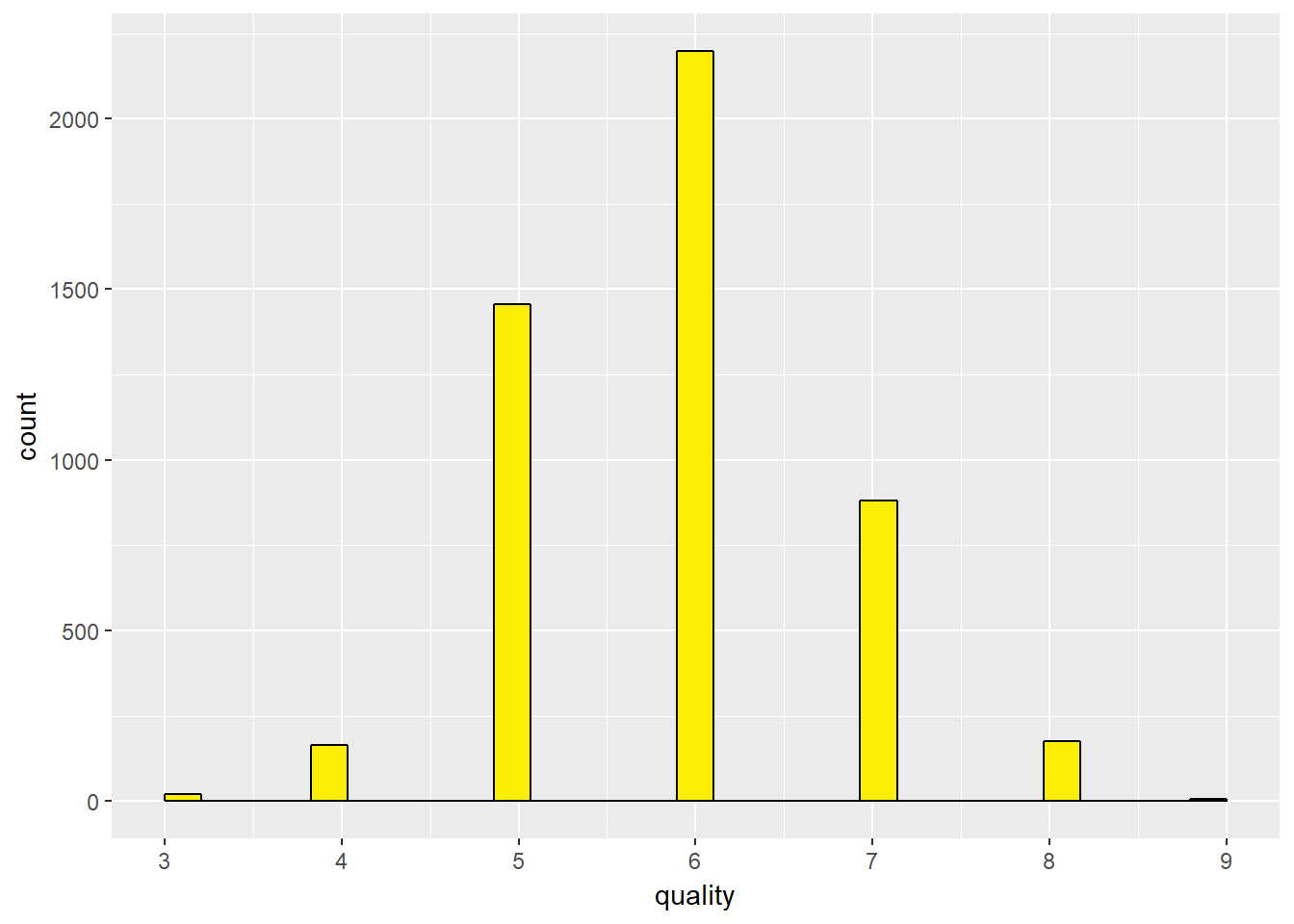``````df\$quality.bucket <- cut(df\$quality, breaks = c(2,4,6,10), labels = c('Poor','Average','Good'))

df\$quality.bucket <- as.factor(df\$quality.bucket)
qplot(x=quality.bucket, data = df,color = I('black'),fill = I('#FCED04'))``````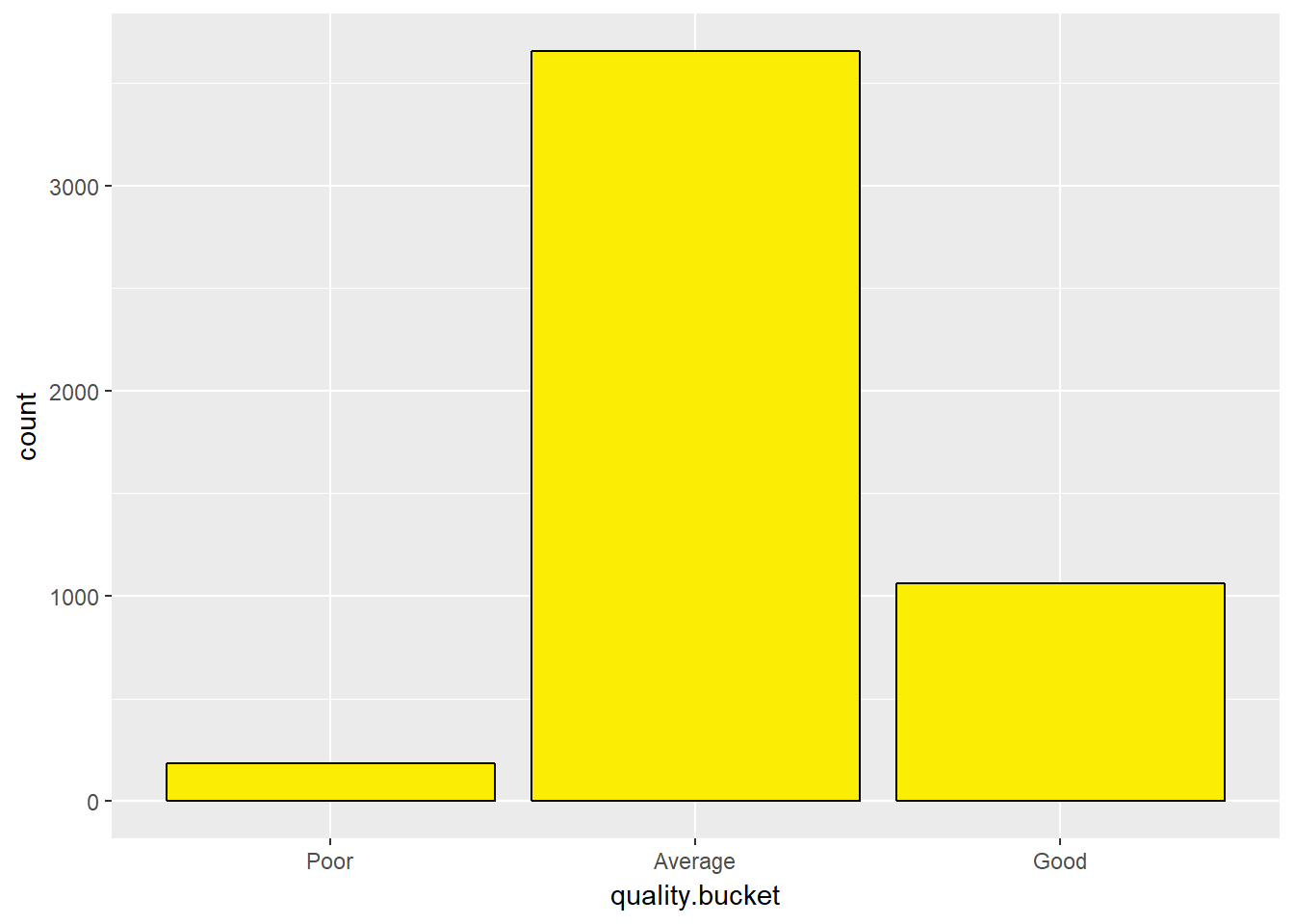Quality of the wine is distributed among 7 levels (3 – 9), 3 being the lowest and 9 being the highest. Most of the wines are of quality 6 which gives the peak of the distribution. Lowest quality(3) and highest quality(9) are very few.

Further, I divided the qualities in 3 categories for simplification of analysis: 1. Poor : Quality 3 to 4 2. Average: Quality 5 to 6 3. Good : Quality 7 to 9

Average wine has the way more majority count in the dataset and Poor wines are very few.

``````ggplot(aes(x = alcohol), data = df)+
geom_histogram(color = I('black'), fill = I('#E80D32'),binwidth = 0.10)+
scale_x_continuous(breaks = seq(8,14,0.5))``````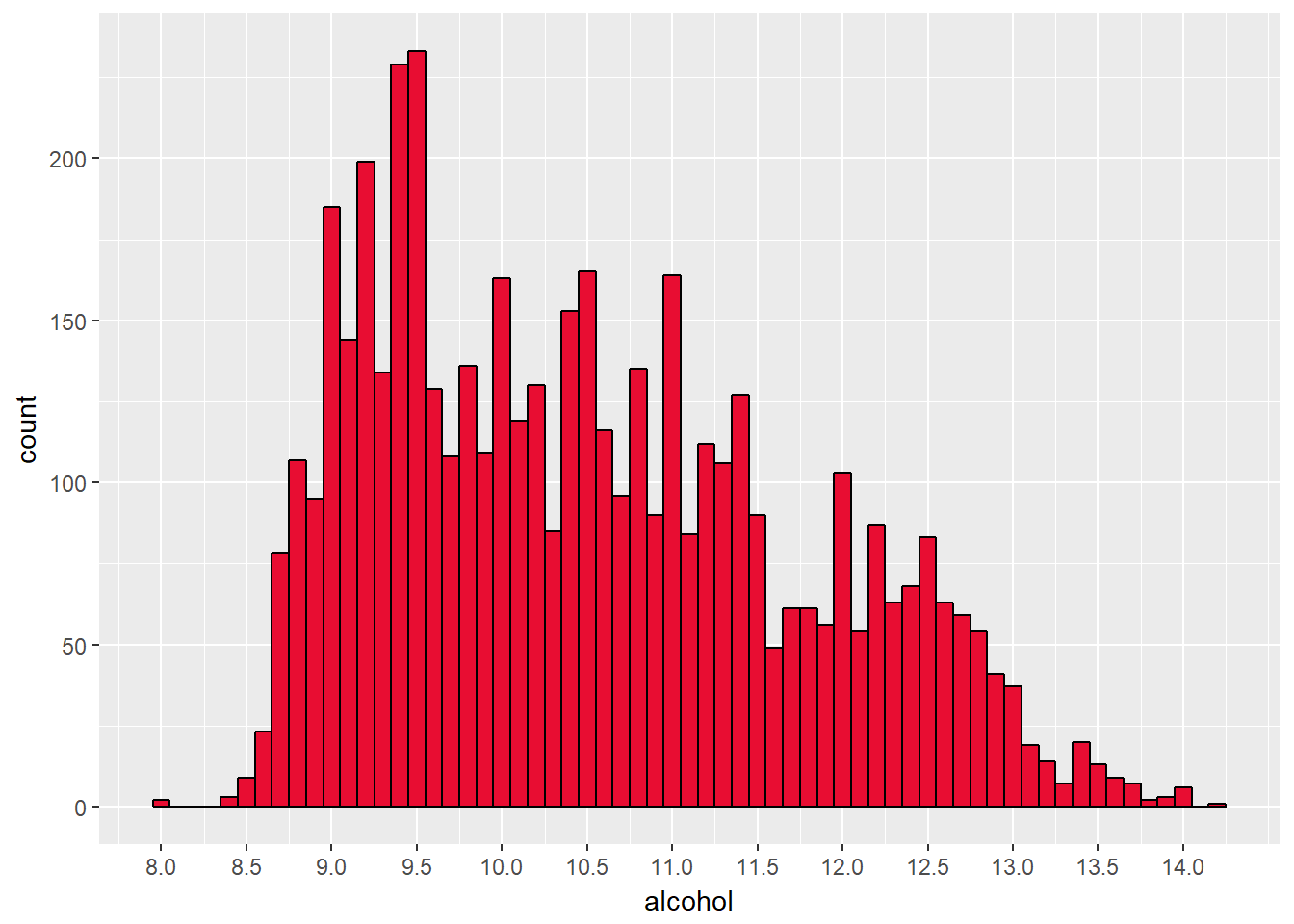``````ggplot(aes(x = alcohol), data = df)+
geom_histogram(color = I('black'), fill = I('#E80D32'))+
facet_wrap(~quality.bucket)``````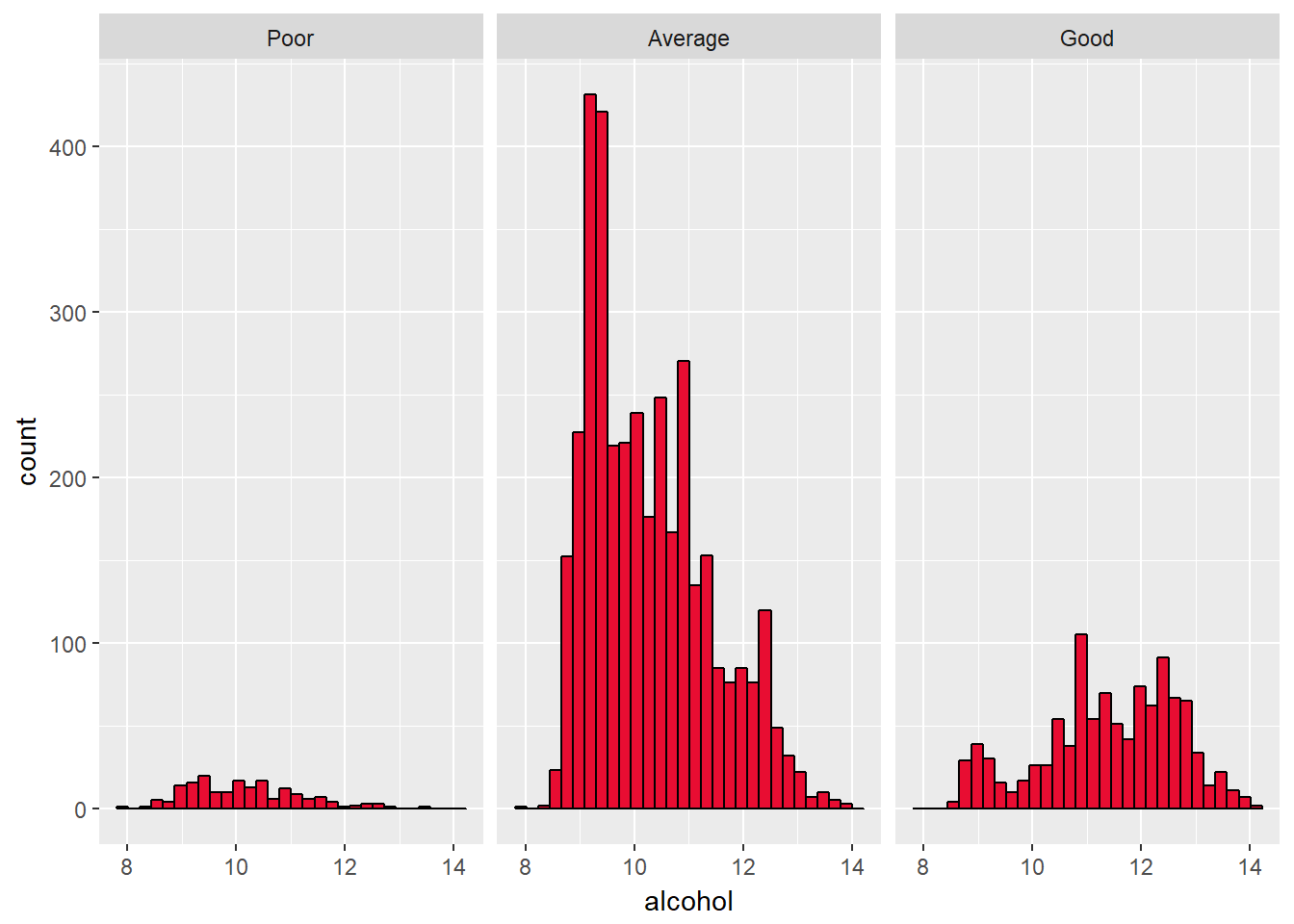The alcohol distribution has a slightly multimodal distribution. Alcohol percentage range between 8 and 14 like most of the alcoholic beverages.

There are peaks between 9 and 9.5 and some peaks between 10 and 11.

Few wines are high alcohol with high alcohol content ( > 12). Very few are with 8% alcohol content.

``````ggplot(aes(x = df\$pH), data = df)+
geom_histogram(color = I('black'), fill = I('#E80D32'),binwidth = 0.1)+
scale_x_continuous(breaks = seq(2.7,3.8,0.1))``````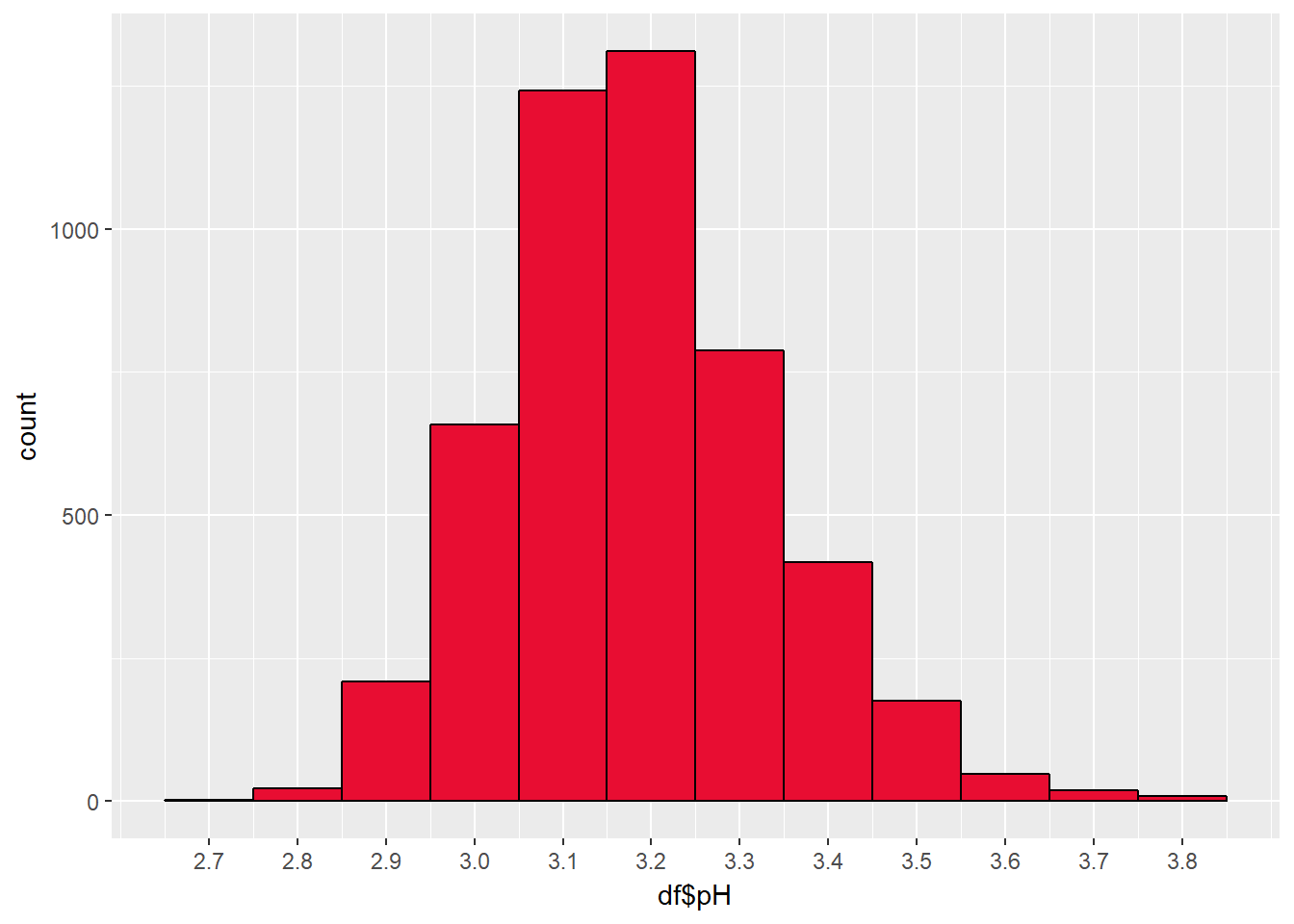``````ggplot(aes(x = pH), data = df)+
geom_histogram(color = I('black'), fill = I('#E80D32'),binwidth = 0.1)+
scale_x_continuous(breaks = seq(2.7,3.8,0.1))+
facet_wrap(~quality.bucket)``````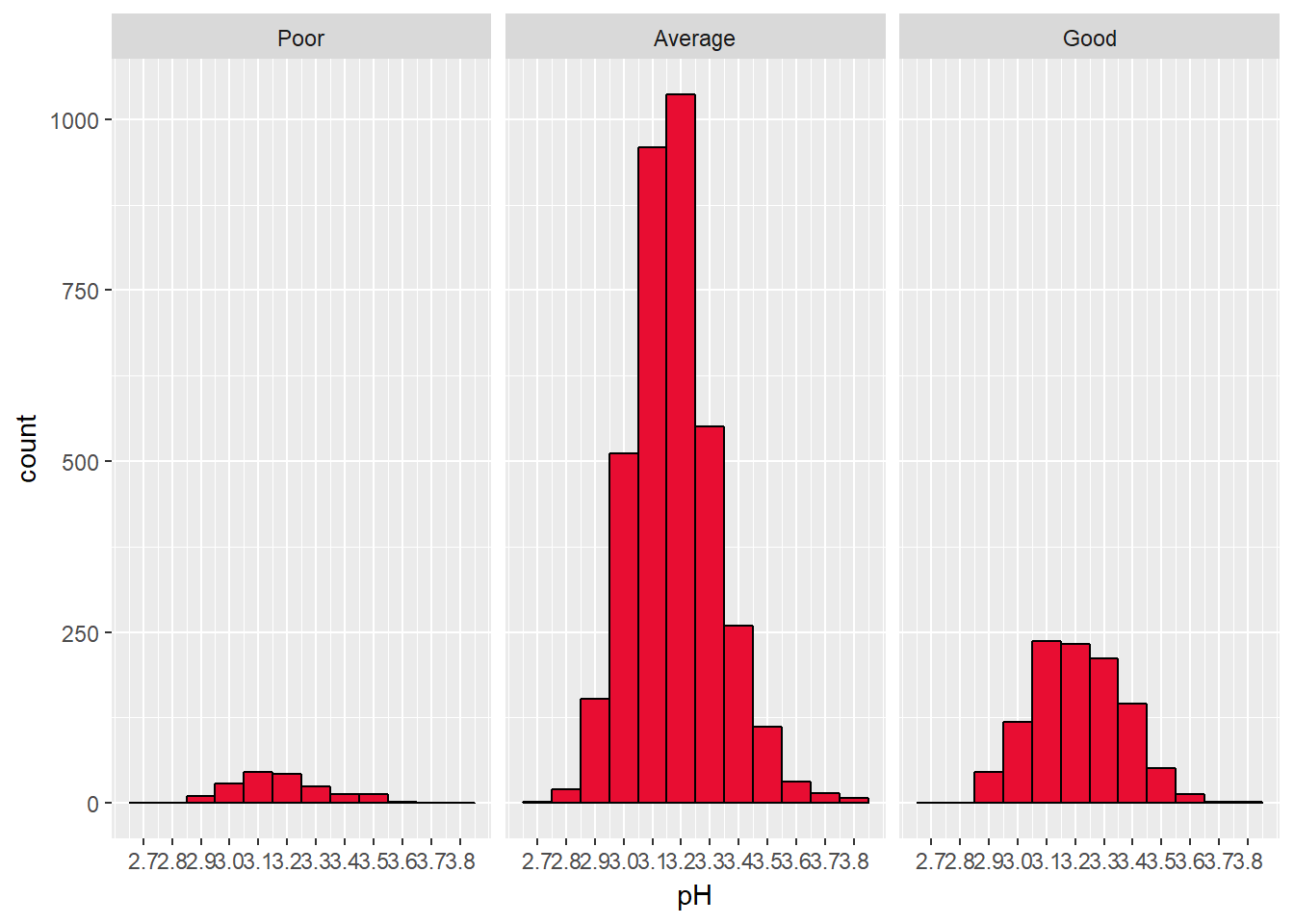Wines are usually acidic in nature. Acidicity is measured by pH scale. Anything having pH below 7 is termed as acidic.

pH distribution of the data set is nearly normal with peak at 3.2, ranging from 2.7 and 3.8

``````ggplot(aes(x = volatile.acidity), data = df)+
geom_histogram(color = I('black'), fill = I('#E80D32'))``````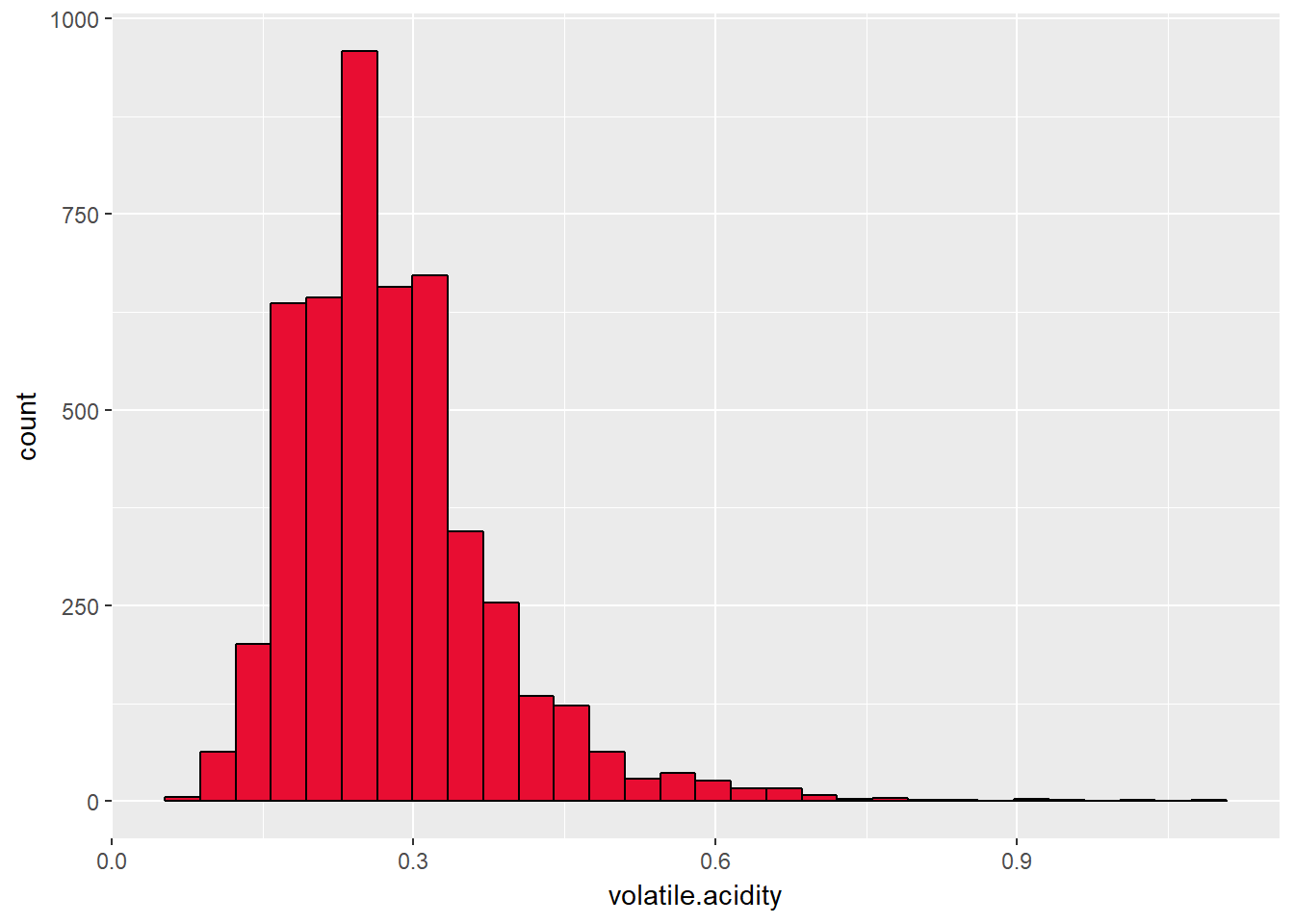``#subset(df, df\$quality == 9)``

Volatile acidity is the amount of acetic acid in wine, which at too high of levels can lead to an unpleasant, vinegar taste. This distribution is right skewed representing fewer wines of high volatile acidity which I think can be a good measure of quality.

``````ggplot(aes(x = residual.sugar), data = df)+
geom_histogram(color = I('black'), fill = I('#E80D32'), binwidth = 0.5)``````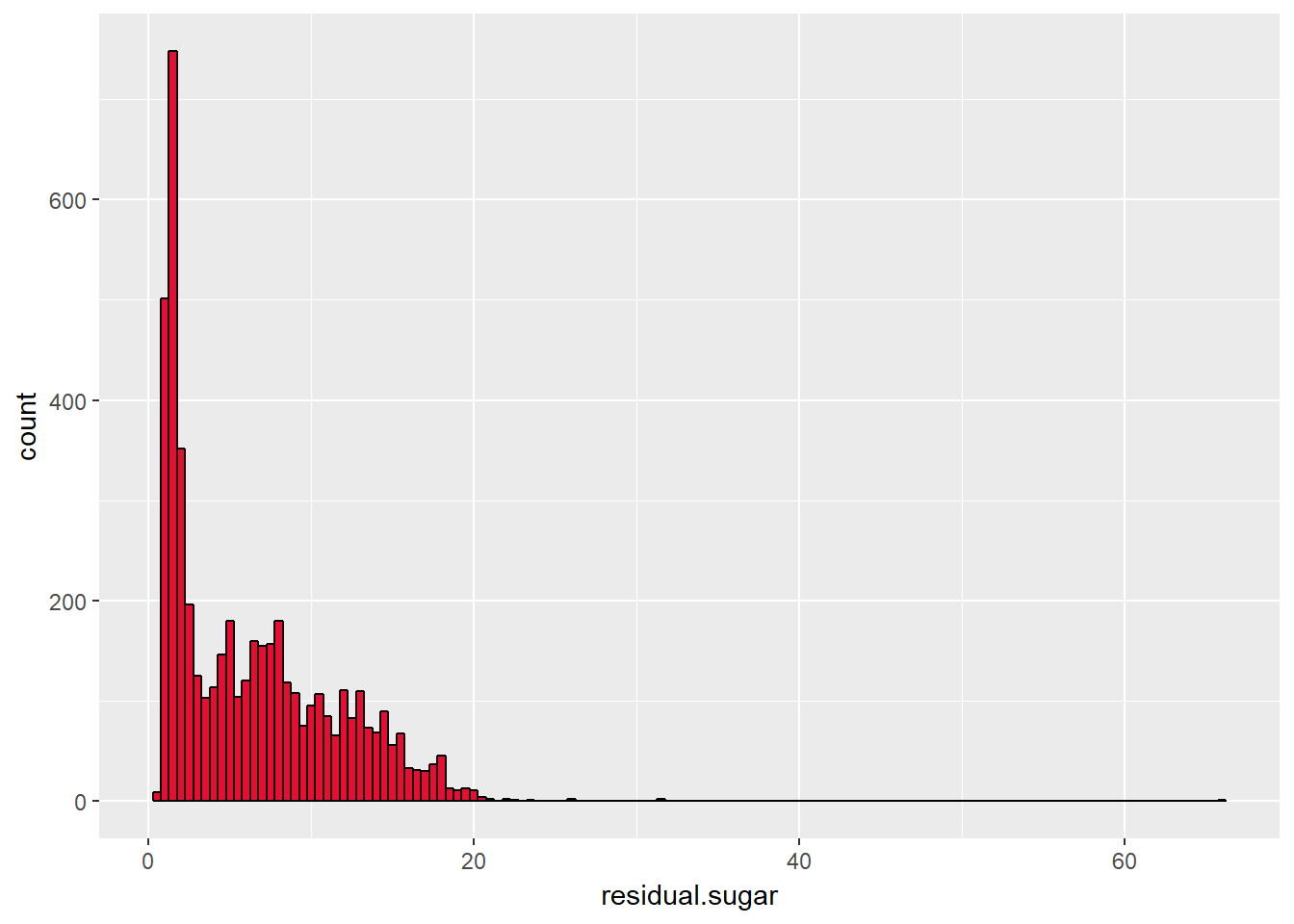``````ggplot(aes(x = residual.sugar), data = df)+
geom_histogram(color = I('black'), fill = I('#E80D32'))+
scale_x_log10()``````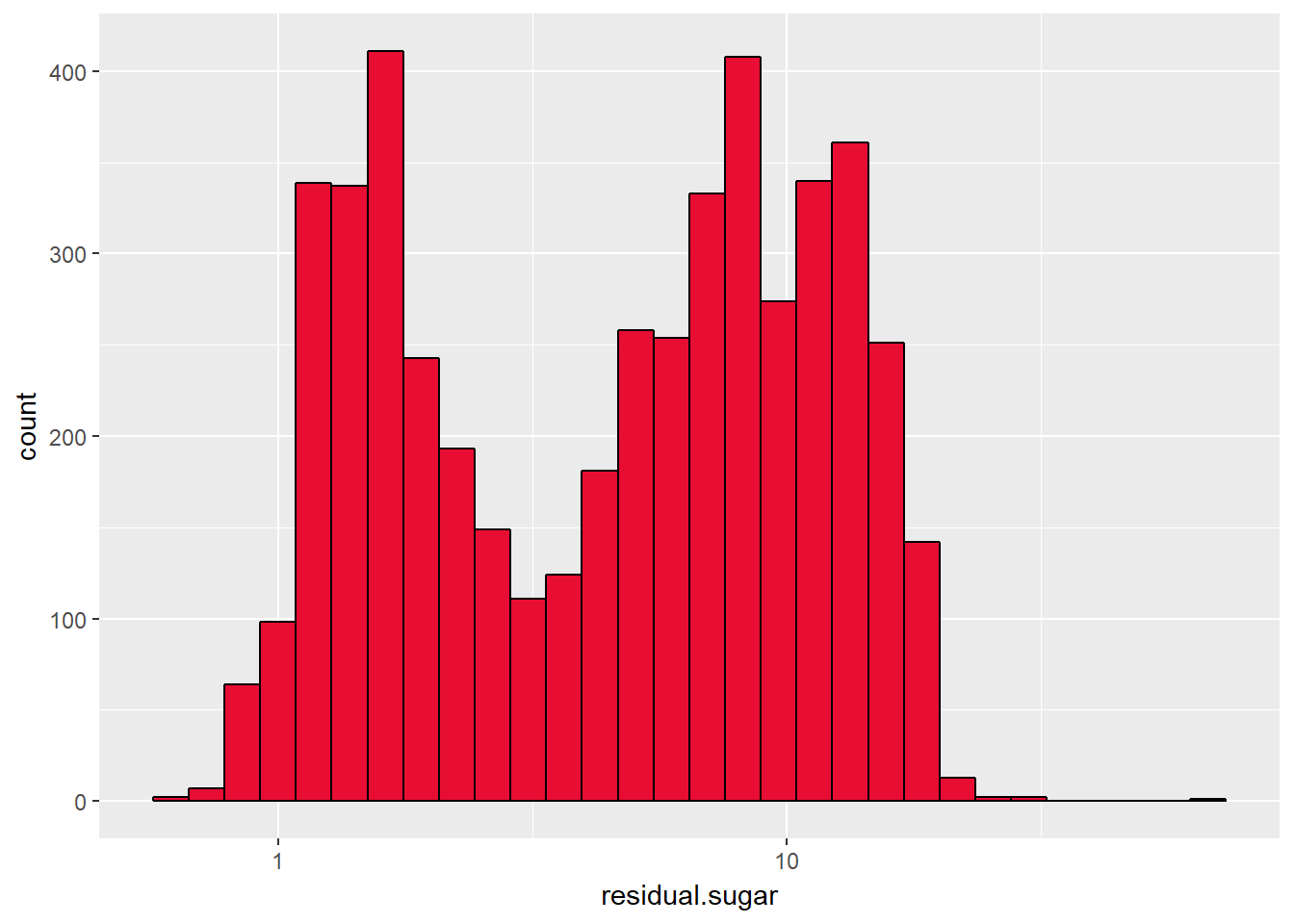``````  ggplot(aes(x = residual.sugar), data = df)+
geom_histogram(color = I('black'), fill = I('#E80D32'))+
facet_wrap(~quality.bucket)``````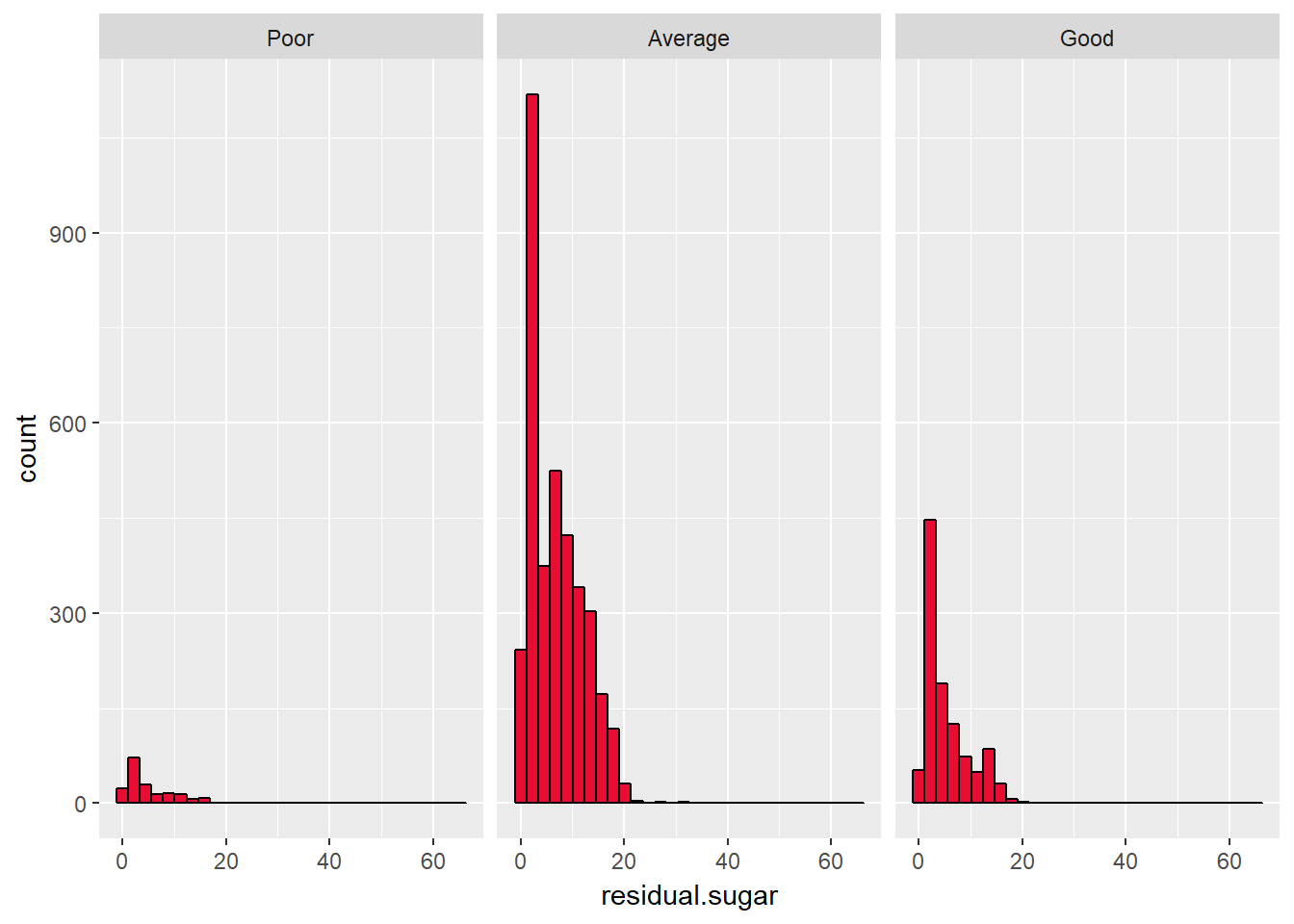The amount of sugar in wine can be a good measure as it can both affect density and alcohol in the wine.

Its distribution is highly right skewed with some outliers. One is at 60.

Transforming the right skewed distribution into log10 scale gives better understanding of the distribution.

The transformed distribution seems bimodal with two peaks at 3 and 10.

``````ggplot(aes(x = density), data = df)+
geom_histogram(color = I('black'), fill = I('#E80D32'), binwidth = 0.00025)+
scale_x_continuous(breaks = seq(0.97,1.00,0.001))+
xlim(c(quantile(df\$density,0.00),quantile(df\$density,0.99)))``````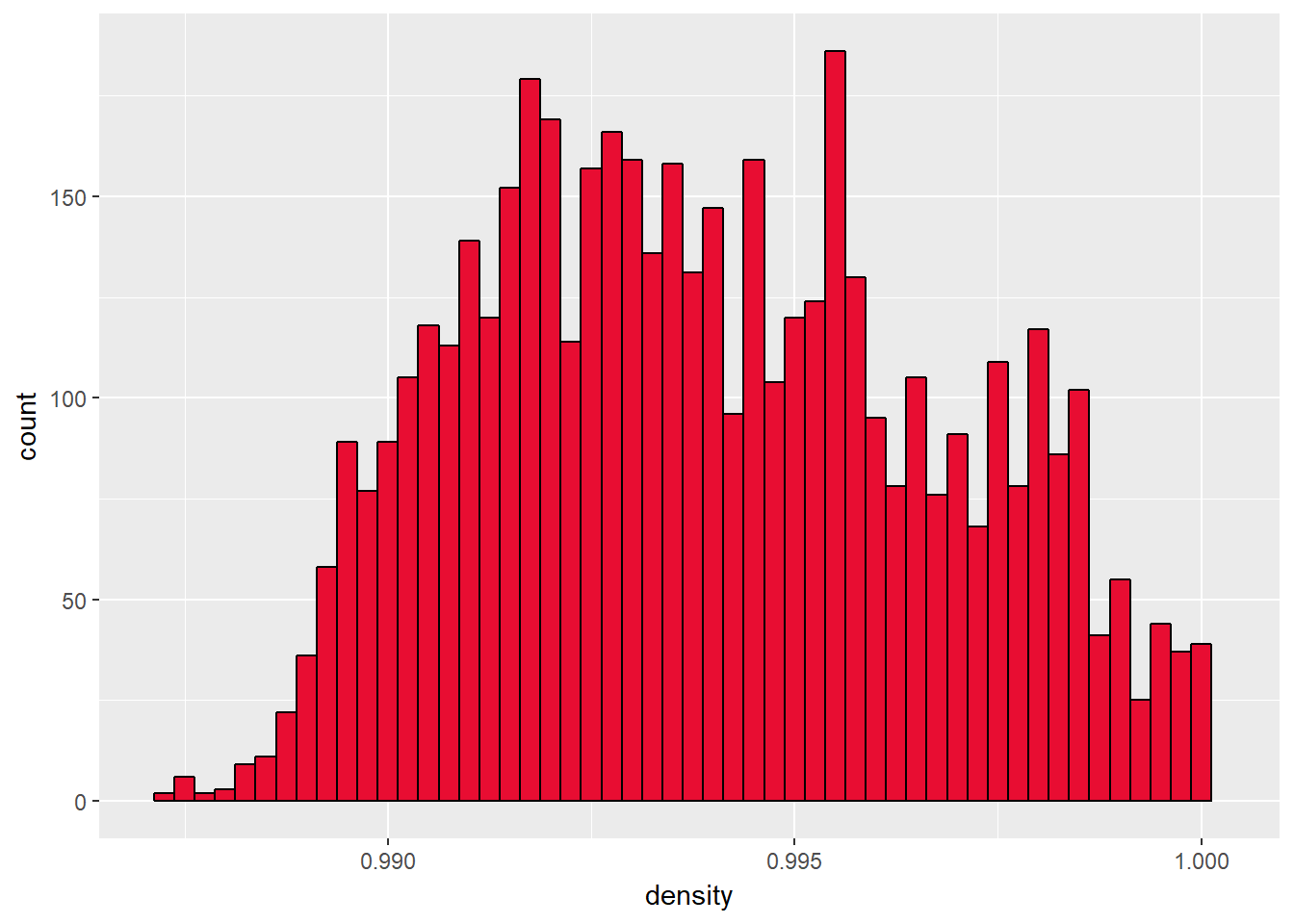Density has some outliers are the higher end near 1.04. That can be because of outlier of residual sugar near 60.

Density’s distribution peaks at 0.995 and majority range between 0.9 and 1

``````ggplot(aes(x = chlorides), data = df)+
geom_histogram(color = I('black'), fill = I('#E80D32'), binwidth = 0.001)+
xlim(c(0,quantile(df\$chlorides,0.95)))``````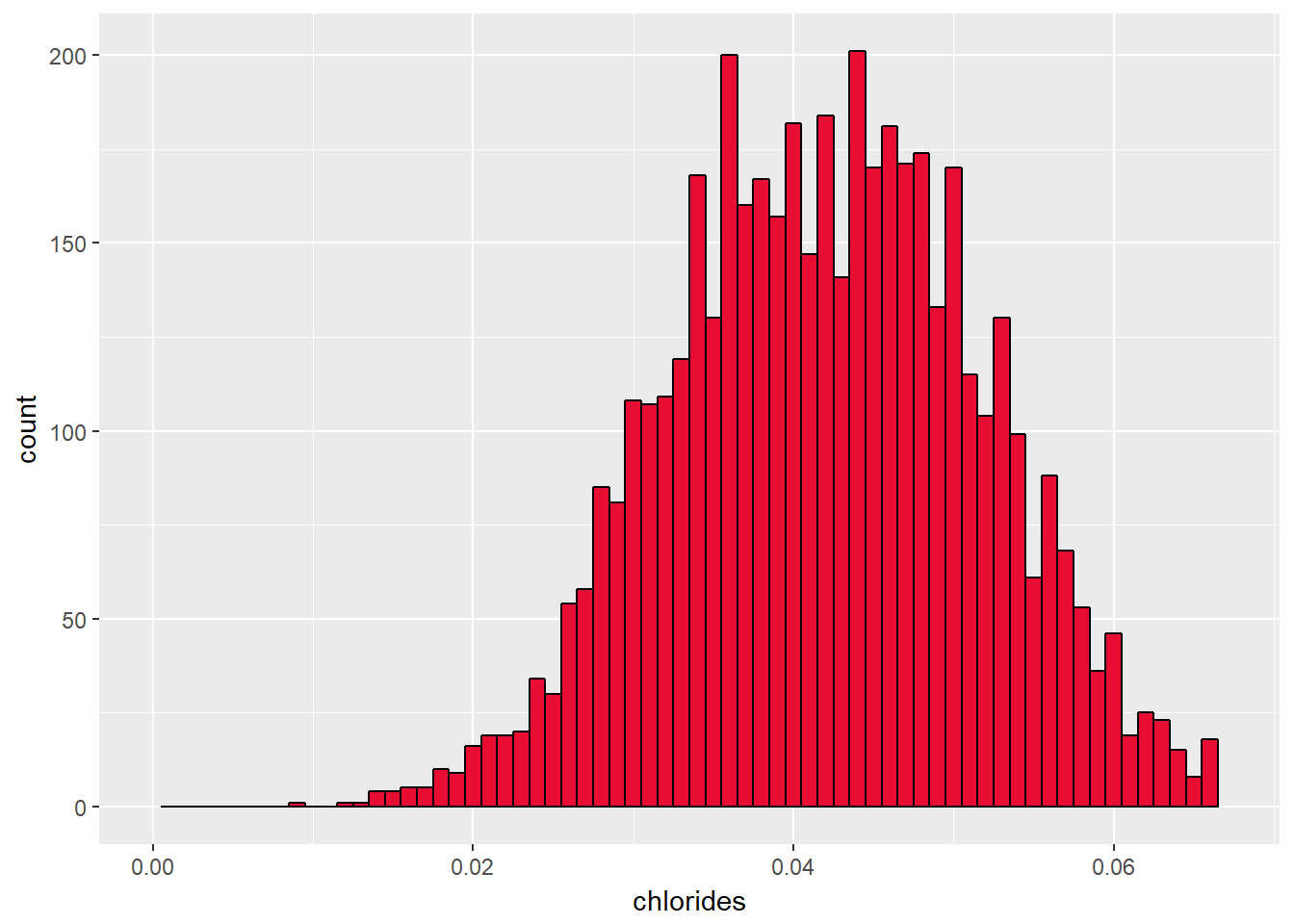Chlorides represent amount of salt in the wine. Majority values are between 0 and 0.3 and peaks at 0.5. It has some outliers at a higher end too.

``````ggplot(aes(x = total.sulfur.dioxide), data = df)+
geom_histogram(color = I('black'), fill = I('#E80D32'), binwidth = 10)+
scale_x_continuous(breaks = seq(0,450,50))``````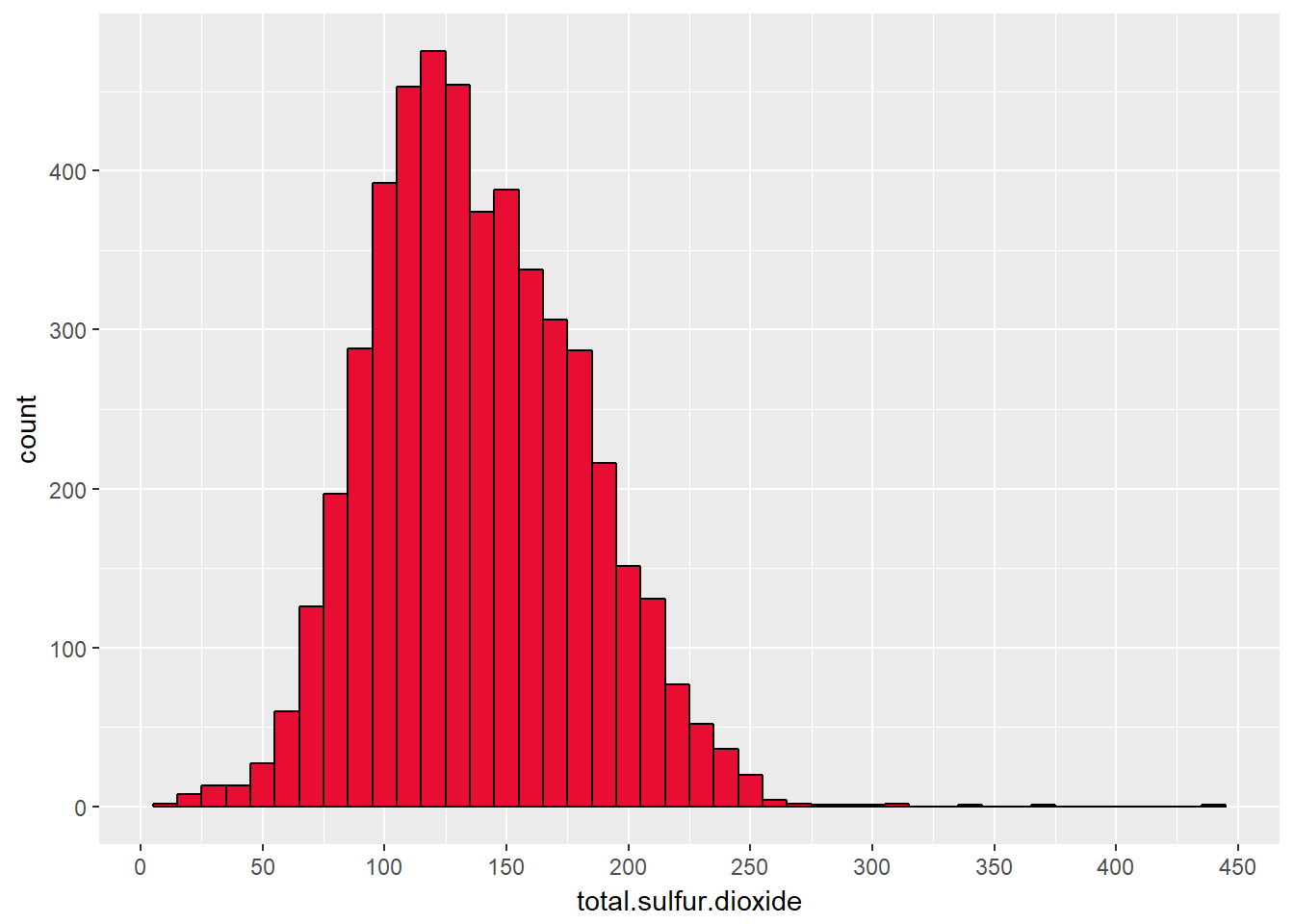Total SO2 is the amount of free and bound forms of SO2.Distribution has some outliers at higher end near 450 and it peaks near 125.

``````ggplot(aes(x = df\$free.sulfur.dioxide), data = df)+
geom_histogram(color = I('black'), fill = I('#E80D32'), binwidth = 5)+
scale_x_continuous(breaks = seq(0,300,30))``````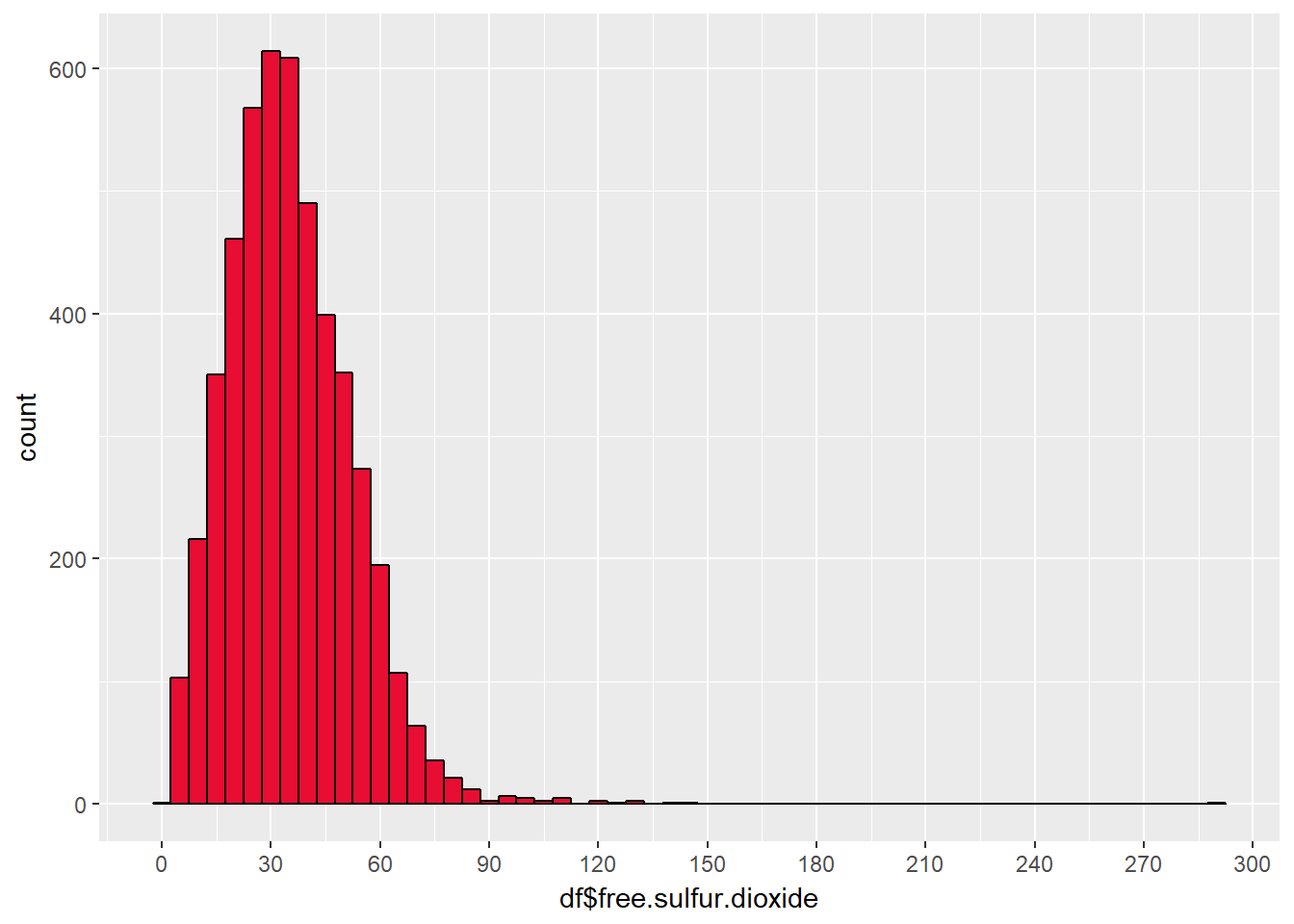Free SO2 concentrations over 50 ppm, SO2 becomes evident in the nose and taste of wine. (Given in variable description). Distribution of Free SO2 is nearly normal with some outliers at the higher end. It peaks just below 45 which I think is good for quality of wine.

``````df\$bound.sulfur.dioxide <- df\$total.sulfur.dioxide-df\$free.sulfur.dioxide

ggplot(aes(x = bound.sulfur.dioxide), data = df)+
geom_histogram(color = I('black'), fill = I('#E80D32'), binwidth = 5)+
scale_x_continuous(breaks = seq(0,300,30))``````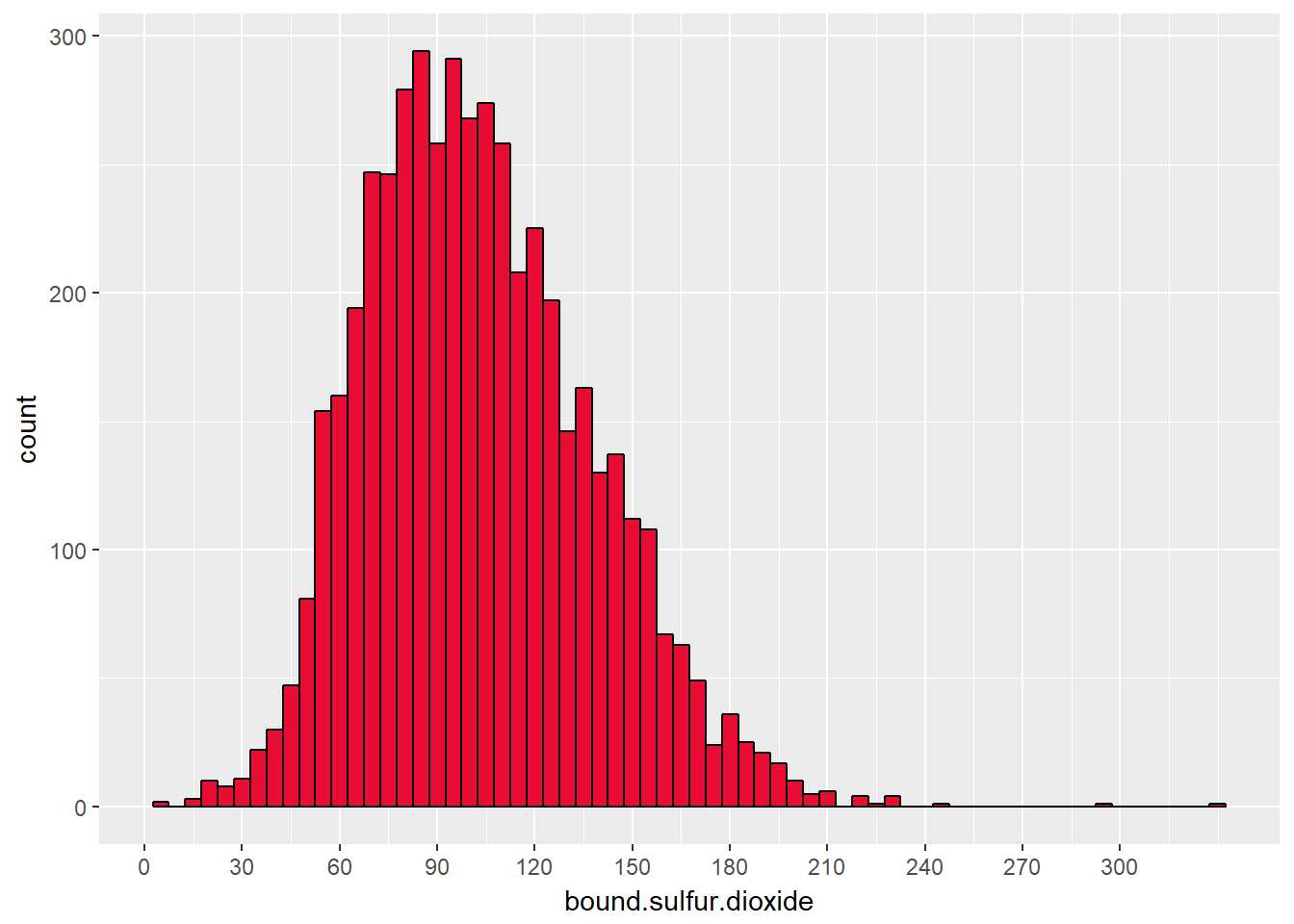I made a new variable that can be of importance in analysing quality of wine.

Bound SO2 = Total SO2 – Free S02

Distribution is pretty much normal with some outliers. I have yet no idea how it contributes to quality. It peaks at 75 and 105.

``````ggplot(aes(x = df\$fixed.acidity), data = df)+
geom_histogram(color = I('black'), fill = I('#E80D32'), binwidth = 0.2)+
scale_x_continuous(breaks = seq(0,15,1))``````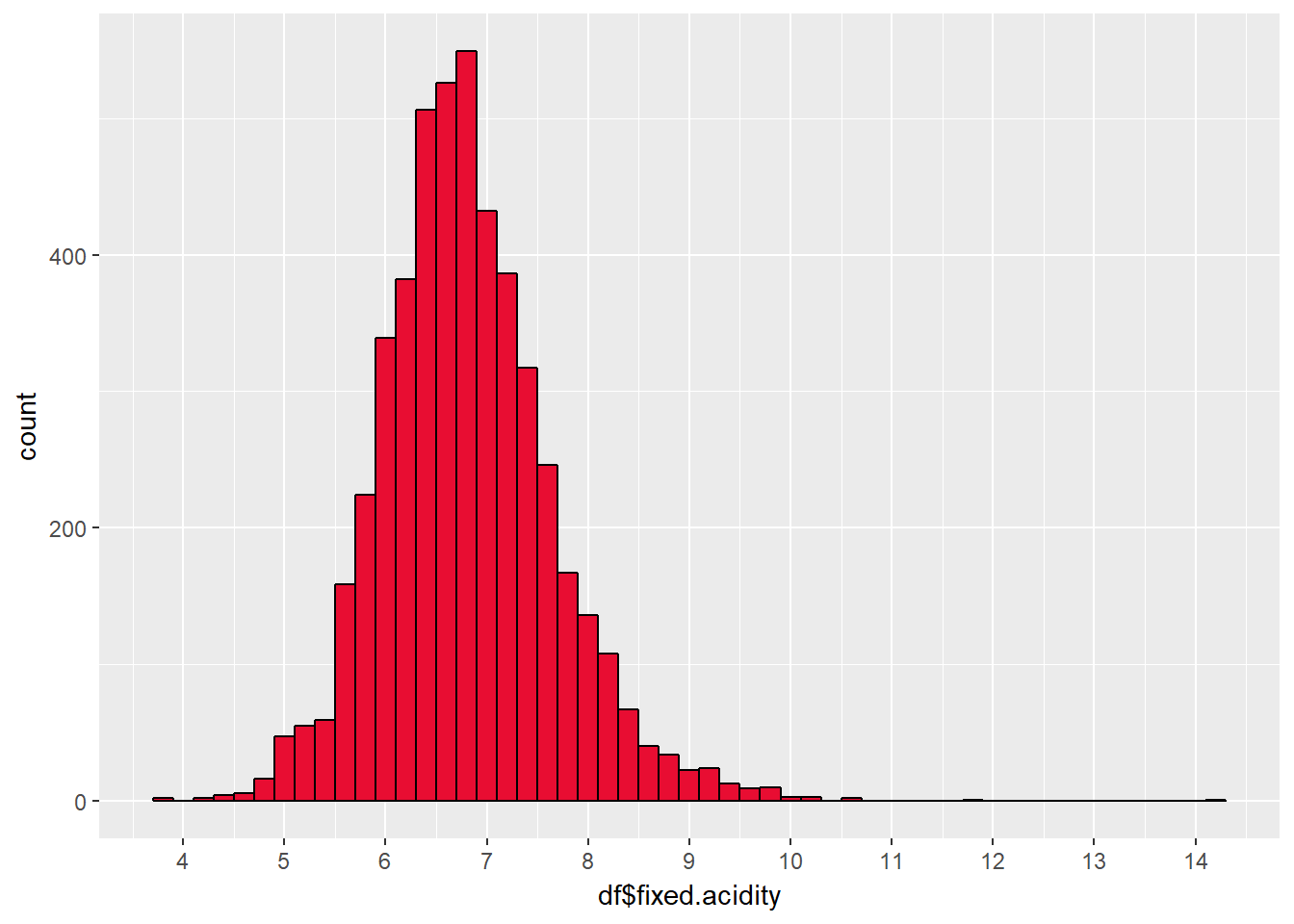Nearly normal distribution with some outliers at both ends. Fixed acidity may contribute to pH along with volatile acidity.

It peaks at 7 and most values range between 5 and 9

``````ggplot(aes(x = citric.acid), data = df)+
geom_histogram(color = I('black'), fill = I('#E80D32'), binwidth = 0.025)+
scale_x_continuous(breaks = seq(0,1.5,0.1))``````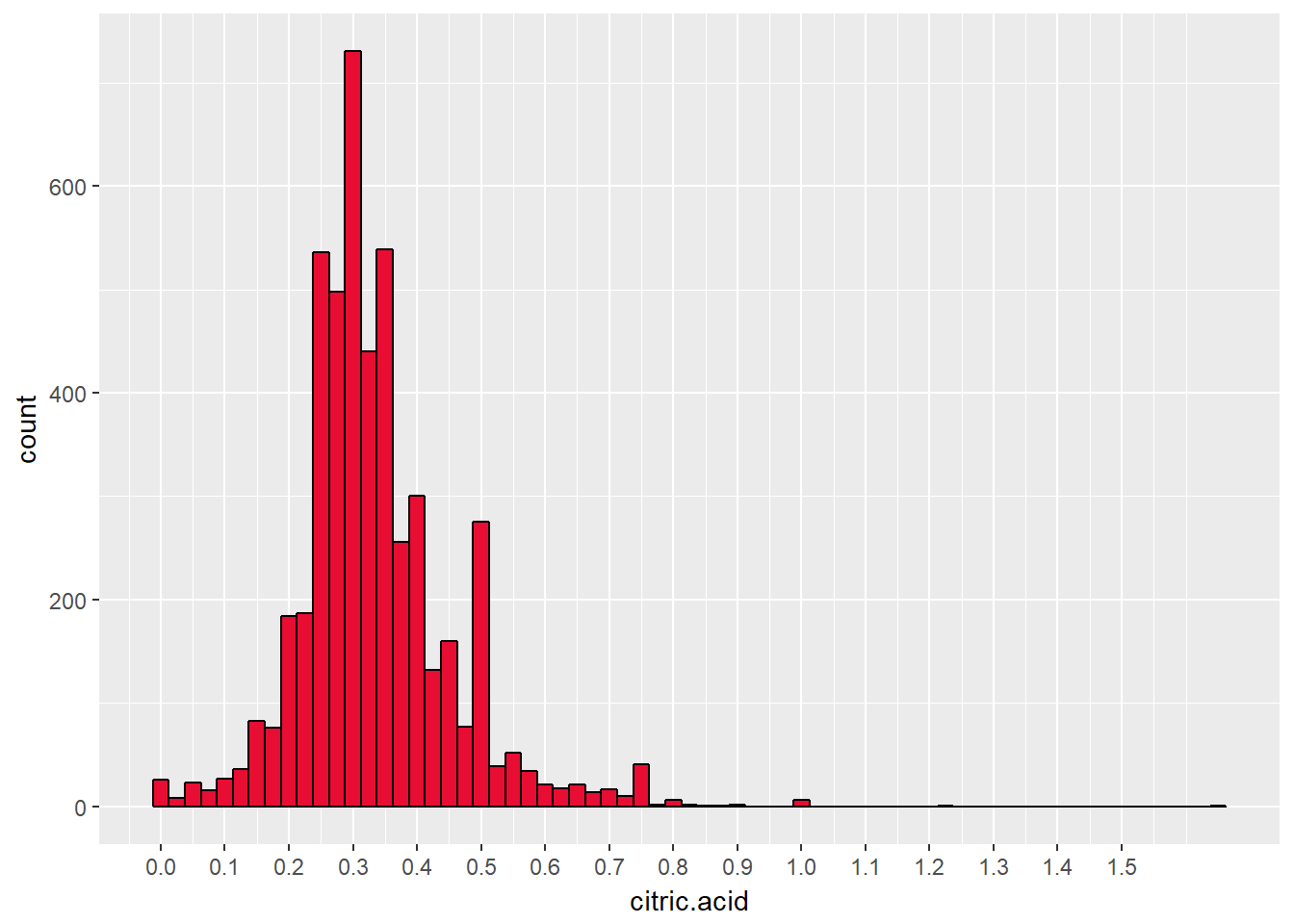Citric acid adds freshness to wine. So, I think in decent amounts its good for quality of wine. It has some outliers and peaks at 0.3

## Let’s now summarise our above analysis by asking ourselves some important questions!

### What is the structure of our dataset?

It has 4898 observation with 13 variables.

### What is/are the main feature(s) of interest in our dataset?

Quality is the main feature of interest. And we think alcohol distribution is interesting too.

### What other features in the dataset do we think will help support us into our feature(s) of interest?

Density, Alcohol, Residual Sugar will definitely help us. We can feel some relationship between them. SO2 levels and PH can be of help.

### Did we create any new variables from existing variables in the dataset?

Yes, we created two variables. One is “quality.bucket” which categorizes quality into poor, average and good. The other is “bound.sulphur.dioxide’ which is a submission of total SO2 and Free SO2.

### Of the features we investigated, were there any unusual distributions? we perform any operations on the data to tidy, adjust, or change the form the data? If so, why did we did this?

Yes, we transformed residual.sugar distribution to log10 as it was highly high skewed. Log10 transformation helps in better understanding as it changes the distribution to nearly normal. Moreover, we transformed various scales and put limitations on x scale for 95 percentile to discard outliers for a better understanding of the plots.

Comment below for any doubt. Next up is Bivariate Analysis!

Share this article!

### Akshay Chaudhary

"If Information is the oil of the 21st century then analytics is the combustion engine”
A senior year engineering student on his learning adventure to become a Data Scientist.### Akshay Chaudhary

"If Information is the oil of the 21st century then analytics is the combustion engine” A senior year engineering student on his learning adventure to become a Data Scientist.

### 3 Comments

1.sayeed alvi

why have you not tried to explain the commands used to do the analysis?

•Akshay Chaudhary

Hi Sayeed!
The basic motive behind the post was to introduce audience to EDA and it’s concepts.
EDA is an art of asking questions and answering at the same time. This is what we focused on!
I used R ggplot2 lib , you can have your own choice to perform EDA
Moreover, the coding or commands used are pretty understandable from the ggplot2 documentation.
I hope you liked the post 🙂

2.Yash

“bound.sulphur.dioxide’ which is a submission of total SO2 and Free SO2.
Is this statement correct?